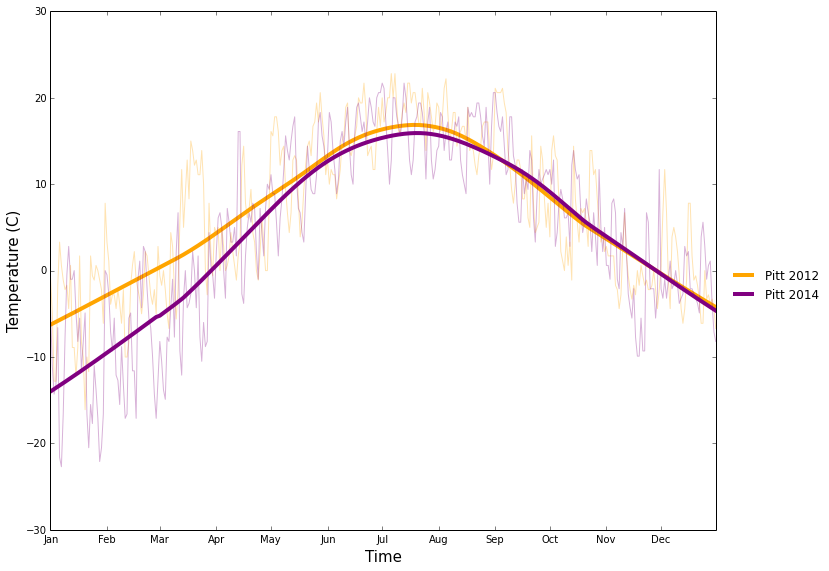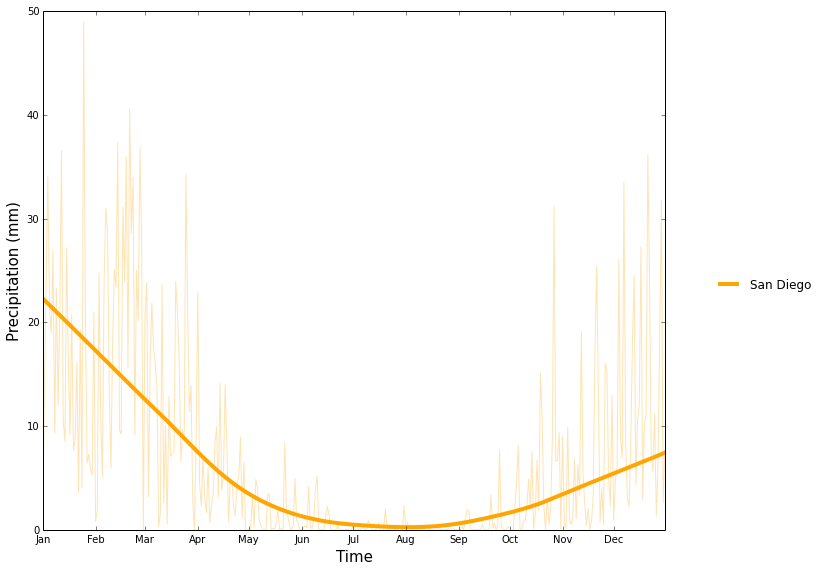# Global Historical Climatology Network data¶

The Global Surface Network (GSN) is a network of weather observatories that continuously collect high quality weather data. The front page for the repository is here:

http://www.ncdc.noaa.gov/cdo-web/datasets

The dataset includes measurements of temperature, precipitation, and many other variables at a large number of observation stations. The data are available in annual, monthly, and daily summaries. We will use the daily summaries here.

The data are available from ftp://ftp.ncdc.noaa.gov/pub/data/ghcn/daily, the file is called "ghcnd_gsn.tar.gz". I unpacked this archive into a directory called ghcnd_gsn in the working directory, then gzipped the individual files to save space.

See the file readme.txt on the ftp site for a discussion of the file formats.

There are many things that can be done with these data. Below we implement functions that allow us to overlay the mean series for any variable of interest (e.g. daily minimum temperature) from any set of locations.

We first need the standard imports.

In :
import numpy as np
import pandas as pd
import statsmodels.api as sm
import os


The data are in separate files for each station. The file names have the format ghcnd_gsn/CHM00056571.dly where CHM00056571 is the name of the station. In the next cell, we get all the file names as a list.

In :
hcn_stations = os.listdir("ghcnd_gsn")
print(len(hcn_stations))
print(hcn_stations[0:5])

873
['BR047403040.dly.gz', 'RSM00034866.dly.gz', 'IN005090100.dly.gz', 'AY000890020.dly.gz', 'MZ000067215.dly.gz']


Next we process the file names to include only the actual name of the station.

In :
hcn_stations = [x.replace(".dly.gz", "") for x in hcn_stations]


Just in case, we check to make sure that every station is represented by a single data file.

In :
print(len(hcn_stations))
print(len(set(hcn_stations)))
print(hcn_stations[0:5])

873
873
['BR047403040', 'RSM00034866', 'IN005090100', 'AY000890020', 'MZ000067215']


The file archive also contains a station meta-data file called ghcnd-stations.txt, which links each station identifier to a place name and geospatial coordinates (longitude and latitude). We create a pandas data frame containing this meta-data about each of the stations. The meta-data file is in fixed-width format, so we located the field boundaries manually.

In :
fname = "ghcnd-stations.txt"
colspecs = [(0, 11), (12, 20), (21, 30), (41, 71)]
station_info = pd.read_fwf(fname, colspecs=colspecs, header=None)
station_info.columns = ["id", "latit", "longit", "name"]
print(station_info.dtypes)
print(station_info.head())
print(station_info.shape)

id         object
latit     float64
longit    float64
name       object
dtype: object
id    latit   longit                   name
0  ACW00011604  17.1167 -61.7833  ST JOHNS COOLIDGE FLD
1  ACW00011647  17.1333 -61.7833               ST JOHNS
2  AE000041196  25.3330  55.5170    SHARJAH INTER. AIRP
3  AF000040930  35.3170  69.0170           NORTH-SALANG
4  AG000060390  36.7167   3.2500     ALGER-DAR EL BEIDA
(92040, 4)


The stations index file contains records for many stations for which we do not have any actual data. Due to the way we are using these data below, we want to remove these stations from station_data. There are several ways to do this. The next cell contains one approach that should be quite efficient.

In :
station_info = station_info.set_index("id")
station_info = station_info.reindex(hcn_stations)
print(station_info.shape)
print(station_info.head())

(873, 3)
latit  longit       name
id
BR047403040 -12.150 -45.000  BARREIRAS
RSM00034866  46.200  45.400     JASKUL
IN005090100  22.367  69.083     DWARKA
AY000890020 -70.667  -8.250   NEUMAYER
MZ000067215 -12.983  40.533      PEMBA


The following function allows us to look up the closest station to a given geographic point.

In :
def find_station(longit, latit):
dx = (latit - station_info.latit)**2 + (longit - station_info.longit)**2
idx = np.argmin(dx)
return station_info.loc[idx, :]


This is how we can use the find_stations_loc function to find the closest station to Ann Arbor, Michigan:

In :
id_aa = find_station(-83, 42)
print(id_aa)

latit                40.4847
longit              -80.2144
name      PITTSBURGH INTL AP
Name: USW00094823, dtype: object


Here is a function that retrieves all the data from a given station, for a given climate variable, in a given year. The data are returned as a Pandas Series object containing the requested data. The series' index is the date, with the year set arbitrarily to 2000 so we can overplot the series for different years.

In :
def get_station_data(stn_id, vtype, year):
"""
Retrieve data from a given station.

Parameters:
-----------
stn_id : string
Station id
vtype : string
Type of measurement to return (one of TMIN, TMAX, PRCP)
year : integer
Year for which to retrieve data
"""

# The data are stored in a tar archive.
fname = os.path.join("ghcnd_gsn", stn_id + ".dly.gz")

# Define the file layout
colspecs = [(17, 21), (11, 15), (15, 17)]
colspecs.extend([(8*k+22, 8*k+27) for k in range(31)])
columns = ["Type", "Year", "Month"] + [d for d in range(1, 32)]

# Read the fixed width file for the requested station
data = pd.read_fwf(fname, colspecs=colspecs, header=None, na_values=9999, compression="gzip")
data.columns = columns
data = data[data["Type"] == vtype]
data = data[data["Year"] == year]

# Melt the days from columns into rows
data = pd.melt(data, id_vars=["Type", "Year", "Month"], var_name="Day", value_name=vtype).dropna()

# Build a date variable but use a fake year so we overplot distinct years
data["Date"] = data.apply(lambda x : "2000-%d-%d" % (x.Month, x.Day), axis=1)
data["Date"] = pd.to_datetime(data["Date"], format="%Y-%m-%d")

if vtype in ("TMIN", "TMAX"):
# Temperatures are stored in tenths of degree C
data[vtype] = data[vtype].astype(np.float64) / 10
else:
# '0T' means 'trace', we want to plot numbers so convert it
data[vtype] = data[vtype].replace({"0T": 0})
data[vtype] = data[vtype].convert_objects(convert_numeric=True)

data = data.set_index("Date")
data = data.drop(["Year", "Month", "Day", "Type"], axis=1)
data = data.sort_index()
data = data[vtype]

return data


Here we retrieve the data for two years at one station.

In :
pitt_2012 = get_station_data(id_aa.name, "TMIN", 2012)
pitt_2014 = get_station_data(id_aa.name, "TMIN", 2014)


Here is a function that we can use to overlay several annual data sequences. The raw data and a smoothed curve are plotted for each series.

In :
def plot_annual(series, labels, frac=0.66, alpha=0.3, add_smooth=True):
colors = ['orange', 'purple', 'lime']
plt.figure(figsize=(12,8))
ax = plt.axes([0.1, 0.1, 0.77, 0.9])
for i, (x, lab) in enumerate(zip(series, labels)):
plt.plot(x.index, x.values, color=colors[i], alpha=alpha)
if add_smooth:
lw = sm.nonparametric.lowess(x.values, np.arange(len(x.values)), frac=frac)
plt.plot(x.index, lw[:, 1], label=lab, color=colors[i], lw=4)
ha, lb = plt.gca().get_legend_handles_labels()
leg = plt.figlegend(ha, lb, "center right")
leg.draw_frame(False)
plt.xlabel("Time", size=15)
if series.name in ("TMIN", "TMAX"):
plt.ylabel("Temperature (C)", size=15)
else:
plt.ylabel("Precipitation (mm)", size=15)
ax.set_position([0.1, 0.1, 0.72, 0.9])

from matplotlib.dates import MonthLocator, DateFormatter
months = MonthLocator()
months_fmt = DateFormatter("%b")
ax = plt.gca()
ax.xaxis.set_major_locator(months)
ax.xaxis.set_major_formatter(months_fmt)


Here is how the above function can be used to plot several series.

In [ ]:
plot_annual((pitt_2012, pitt_2014), ("Pitt 2012", "Pitt 2014"), frac=0.4)Next we look at the precipitation data. Precipitation is nearly always more skewed and more variable than temperature. So a single year's precipitation data will not be very informative. Thus take 25 years of precipitation data from San Diego and plot the mean curve.

In [ ]:
id_sd = find_station(-117, 33)
print(id_sd)
sd_data = [get_station_data(id_sd.name, "PRCP", year) for year in range(1990, 2015)]

latit                     32.7336
longit                  -117.1831
name      SAN DIEGO LINDBERGH FLD
Name: USW00023188, dtype: object

In [ ]:
sd_df = pd.DataFrame(sd_data).T
sd_mn = sd_df.mean(1)
plot_annual((sd_mn,), ("San Diego",), frac=0.4)